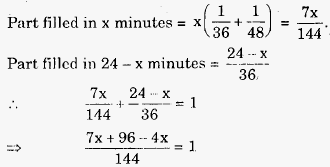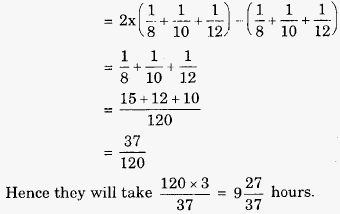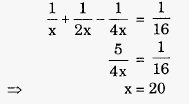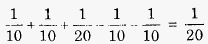# Engineering Maths - Time and Work

>>>>>>>>Time and Work

• A

8 mins.• B

9 mins.• C

12 mins.• D

16 mins.• Option : D
• Explanation :
Let pipe B be turned off after x minutes.⇒ x = 16 minutes

• A

In the 8th hour.• B

In the 9th hour.• C

In the 10th hour.• D

In the 11th hour.• Option : C
• Explanation :
In a cycle of 3 hours: pipes P, Q and R are working as inlet pipes for 2 hours each and they are working as outlet pipes for an hour each. So part of tank filled in 3 hours.Thus, tank will be filled in 10th hour.

• Option : B
• Explanation :
Let number of hours taken by the outlet and the 2 inlet pipes be 4x, 2x and x respectively.
So in 1 hour the part of empty tank filled isHence, inlet pipe with higher efficiency fills the tank in 20 hours.
So in one hour, the desired pipes fillRefer to the data below and answer the questions that follow.
The boiler tank in a chemical factory holds 105 litres. 5 tanks each having one-fifth the capacity of the boiler tank fill in ‘hard water’ at same rates in the boiler tank in 2 hours. The outlet of the two of smaller tanks work as inlet pipes and other two work as outlet and the fifth tank fill in the main ‘boiler’ at half its efficiency.

• A

1 hour• B

5 hour• C

20 hour• D

25 hour• Option : C
• Explanation :
Since 5 tanks of same efficiency fill the boiler in 2 hours each tank individually takes
2 x 5 = 10 hours to fill it.
Tank with half the efficiency will take 10 x 2 = 20 hours.
In one hour, fraction of boiler getting filled when 4 fully efficient pipes with two as inlet and two as outlet and 5th acts at 50% efficiency as an inlet isHence it will take 20 hours to fill the boiler tank.

Refer to the data below and answer the questions that follow.
The boiler tank in a chemical factory holds 105; litres. 5 tanks each having one-fifth the capacity of the boiler tank fill in ‘hard water’ at same rates in the boiler tank in 2 hours. The outlet of the two of smaller tanks work as inlet pipes and other two work as outlet and the fifth tank fill in the main ‘boiler’ at half its efficiency.

• A

2 hours• B

4 hours• C

6 hours• D

8 hours• Option : C
• Explanation :
Here fraction of tank filled in 1 hourth of the tank
If they are opened alternately, then tank gets filled in 4 x 3 = 12 hours
Hence half of the tank gets filled in 12/2 = 6 hours.
Related Quiz.
Time and Work